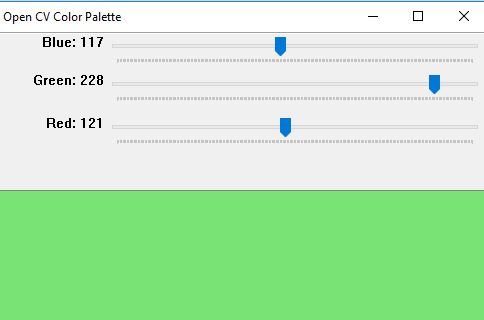# Python | OpenCV BGR color palette with trackbars

OpenCV is a library of programming functions mainly aimed at real-time computer vision.
In this article, Let’s create a window which will contain RGB color palette with track bars. By moving the trackbars the value of RGB Colors will change b/w 0 to 255. So using the same, we can find the color with its RGB values.

```Libraries needed:
OpenCV
Numpy```

Approach:
Create a black window with three color channels with resolution 512 x 512. Then create three ‘B’ ‘G’ ‘R’ trackbars using predefined functions of OpenCV library. Set the values of channels from 0 to 255. Merging the black window with these color trackbars.

 `# Python program to create RGB color   ` `# palette with trackbars  ` ` `  `# importing libraries ` `import` `cv2 ` `import` `numpy as np ` `  `  `# empty function called when ` `# any trackbar moves ` `def` `emptyFunction(): ` `    ``pass` `  `  `def` `main(): ` `     `  `    ``# blackwindow having 3 color chanels ` `    ``image ``=` `np.zeros((``512``, ``512``, ``3``), np.uint8)  ` `    ``windowName ``=``"Open CV Color Palette"` `     `  `    ``# window name ` `    ``cv2.namedWindow(windowName)  ` `      `  `    ``# there trackbars which have the name ` `    ``# of trackbars min and max value  ` `    ``cv2.createTrackbar(``'Blue'``, windowName, ``0``, ``255``, emptyFunction) ` `    ``cv2.createTrackbar(``'Green'``, windowName, ``0``, ``255``, emptyFunction) ` `    ``cv2.createTrackbar(``'Red'``, windowName, ``0``, ``255``, emptyFunction) ` `      `  `    ``# Used to open the window ` `    ``# till press the ESC key ` `    ``while``(``True``): ` `        ``cv2.imshow(windowName, image) ` `         `  `        ``if` `cv2.waitKey(``1``) ``=``=` `27``: ` `            ``break` `         `  `        ``# values of blue, green, red ` `        ``blue ``=` `cv2.getTrackbarPos(``'Blue'``, windowName) ` `        ``green ``=` `cv2.getTrackbarPos(``'Green'``, windowName) ` `        ``red ``=` `cv2.getTrackbarPos(``'Red'``, windowName) ` `         `  `        ``# merge all three color chanels and ` `        ``# make the image composites image from rgb    ` `        ``image[:] ``=` `[blue, green, red] ` `        ``print``(blue, green, red) ` `          `  `    ``cv2.destroyAllWindows() ` ` `  `# Calling main()          ` `if` `__name__``=``=``"__main__"``: ` `    ``main() `

Output:Note: Above programs will not run on online IDE.

Whether you're preparing for your first job interview or aiming to upskill in this ever-evolving tech landscape, GeeksforGeeks Courses are your key to success. We provide top-quality content at affordable prices, all geared towards accelerating your growth in a time-bound manner. Join the millions we've already empowered, and we're here to do the same for you. Don't miss out - check it out now!

Previous
Next